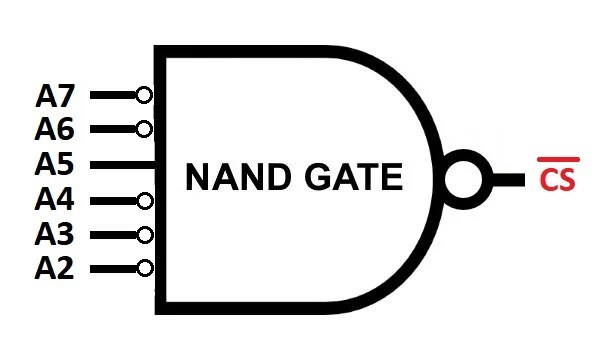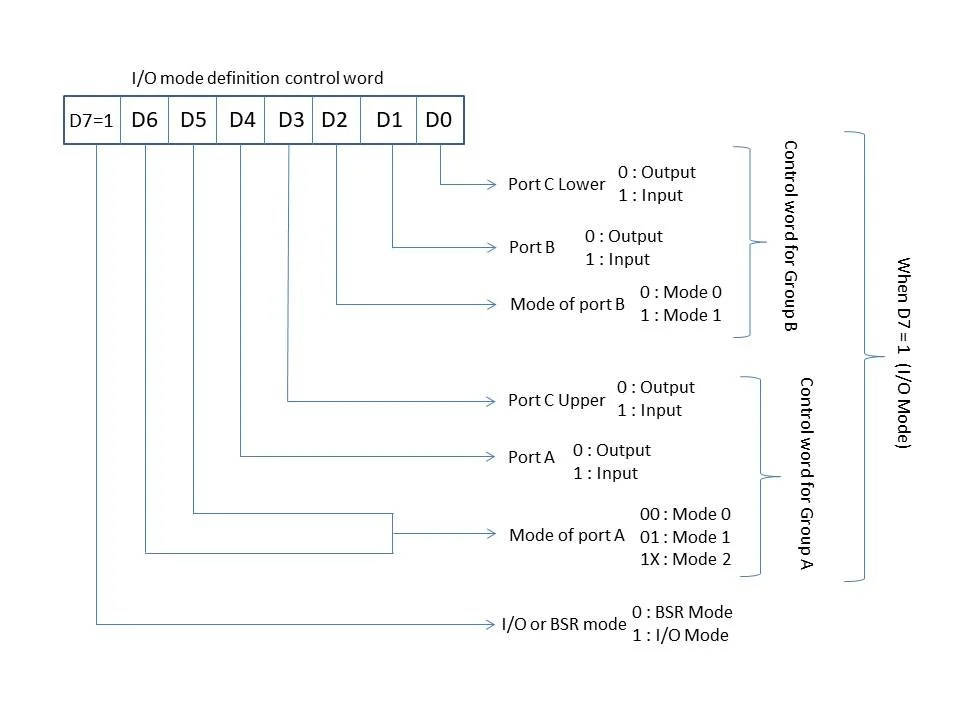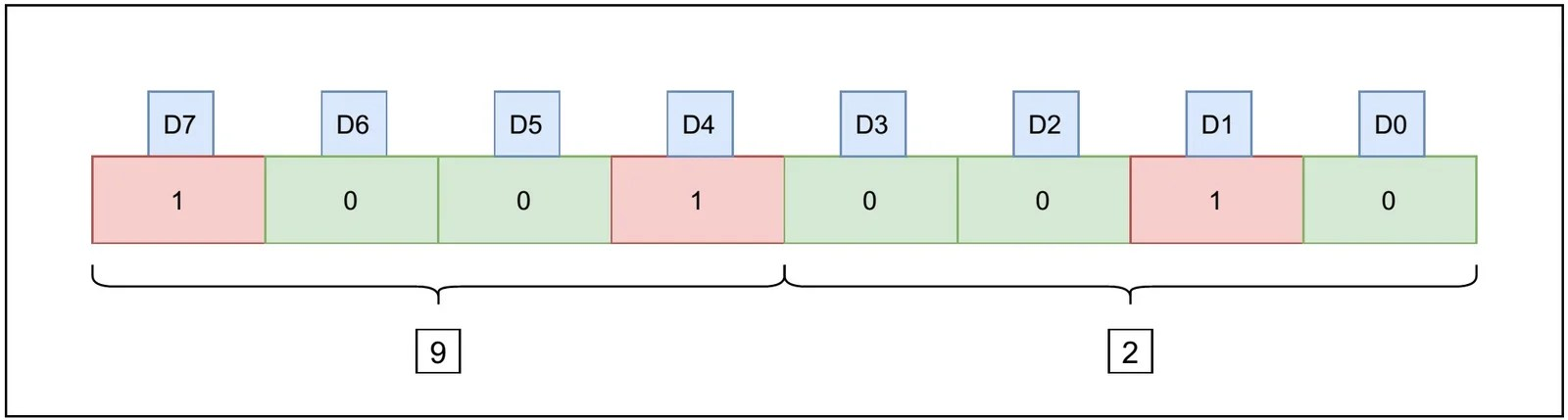View Course Path

# Interfacing of 8051 with 8255 Programmable Peripheral Interface

In this tutorial, we’re going to learn how to interface an 8255 programmable peripheral interface with an 8051 microcontroller. We’ve covered the working of the 8255 PPI in-depth in this guide. Make sure you understand a) the different modes of operation and b) generating the control word of the 8255 before you dive into this tutorial.

Our main aim through this tutorial is to get comfortable with the Chip Select algorithm so that we can extend our knowledge to interface LEDs, as well as, LCDs to 8051 using 8255 PPI. To demonstrate the working after establishing the interface, we’ll take inputs (1 Byte each) from Port A and Port B, add these numbers and send the results to Port C.

Contents

## Why do we need to interface 8255 PPI with 8051?

As you’re familiar with what an 8255 PPI is, let’s bring forward a question. Why do we need additional pins when we have enough on the 8051 already?

For applications that require the pulses from many pins of the 8051, it is always recommended to use 8255 PPI to avoid data loss and damage to the microcontroller.

• Port 0 – Along with the input/output function, this port also serves the function of an address or a data bus (AD0 – AD7).
• Port 2 – Along with I/O operations, it acts as a higher-order address bus while interfacing external memories to 8051.
• Port 3 – This port also has alternate functions like INT0 (P3.2), INT1 (P3.3), RDX, TXD, taking in external pulse from T0, T1, read/write enable pins (P3.6 and P3.7).
• Port 1 – Now, this is the only port with no alternate functions. This is the reason why we need to interface 8255 to provide an additional three ports, namely Port A, Port B, and Port C. And the best part about the 8255 is that it is programmable.

Let’s start off by listing all the components that we require to interface an 8255 PPI with an 8051.

## Components Required

1. 8051 Microcontroller – AT89C51/ C52/ S52 or any other similar variant.
2. 8255 Programmable Peripheral Interface
3. Decoder circuit – 74LS373 (for address demultiplexing)
4. NAND gate IC – 74LS00
5. NOT gate IC – 74LS04

## Chip Select Logic

For a microcontroller to interface with 8255, a LOW pulse is given to the Chip Select pin of 8255 as it is an active low pin (Turns ON when a LOW pulse is fed).

As we don’t want to be constantly connected and only communicate with the 8255 when absolutely needed, we need some form of logic to activate the line connected to the CS pin. Hence, we can design a combinational logic circuit using a NAND gate for which the input will be A2 – A7 address lines and CS as the output. A0 and A1 are mode selector bits.

Since the output of Port 0 consists of both address and the data bus multiplexed together, we need a demultiplexing/ decoder circuit like 74LS373 to separate out the data bus from the address bus, basically convert AD0 – AD7 to A0 – A7.

Let’s allot the address to the ports of 8255 PPI so that we can access them later,

• Port A: 0010 0000 (20H)
• Port B: 0010 0001 (21H)
• Port C: 0010 0010 (22H)
• Control port: 0010 0011 (23H)

Note that here only the last two bits – A0 and A1, are changed so as to select different ports.

The output of the NAND gate is LOW only when ALL the inputs are HIGH. Therefore, from the address allocations that we have done, we have:

• A7 = 0
• A6 = 0
• A5 = 1
• A4 = 0
• A3 = 0
• A2 = 0Therefore, we need to invert the logic of A7, A6, A4, A3, and A2 to input all HIGH logic to the NAND gate. Hence, the combinational logic can be given by

CS = Complement of (A7 + A6 + A5 + A4 + A3 + A2)

NAND (A, B, C) = Complement (A + B + C) – DeMorgan’s theorem

## Algorithm

Based on the following diagram describing all the bits of Control Word register of 8255, let’s create an algorithm to interface 8255 with 8051 to take inputs from Port A and Port B, add them and send results to Port C. For this, we select the value to be given to control word and send it to the control port of 8255.• Step 1: Construct the control word register.
• D7 = 1, this will make sure that all the ports of 8255 PPI are in the I/O mode and not BSR mode.
• D6 = D5 = D2 = 0: selects mode 0 for Port A, Port B, and Port C.
• D4 = D1 = 1: To select Port A and Port B as input ports.
• D3 = D0 = 0: To select both lower and upper Port C as output port.• The control word would be 1001 0010 in binary and 92H in hexadecimal. Now, we write this data to the control port to set the port into desired modes, and that will be it for step 1.
• This is done with the instruction `MOV A, 92H`, and sending this value to location 23H (control register) using `DPTR`.
• Step 2: Input the data from Port A and Port B
• Copy the input from Port A to the Accumulator and save it in the internal RAM in register R0. This is achieved by loading DPTR with 20H (to go to the address of Port A). Receive data from 8255 using `MOVX A, @DPTR` command.
• Copy the input from Port B to the Accumulator and save it in the internal RAM in register R1. This is achieved by loading DPTR with 21H (to go to the address of Port B). Receive data from 8255 using `MOVX A, @DPTR` command.
• Step 3: Add the contents of port A and port B
• Move the contents of R0 into A using `MOV A, R0` command.
• Then add the value in R1 to A using `ADD A, R1` command.
• The result of this addition is in accumulator A.
• Step 4: Display the result in port C
• This is done by loading DPTR with 22H (to go to the address of Port C) and sending the value of accumulator containing the sum using `MOVX @DPTR, A` command.

## Circuit Diagram to interface 8255 PPI with 8051

• Step 1: Connect the power pins of 8255 by connecting VCC and GND pins to the appropriate sources. The EA of 8051 should be connected to VCC as well.
• Step 2: Pins P3.6 (WR) and P3.7 (RD) are to be connected to the WR and RD pins of 8255, respectively. 8051 conveys the type of operation (read or write) to 8255 via these connections.
• Step 3: Connect Port 0 to 74LS373 decoder, i.e. P0.0 – P0.7 to 1D – 8D. You can refer to the figure below to recap the pin diagram of 74LS373. Also, connect the ALE pin of 8051 to the Enable pin (G) of 74LS373 to activate it. You can learn more about demultiplexing address and data lines using 74LS373 here.
• Step 4: Tap the AD0 – AD7 lines of Port 0 and connect it directly to D0 – D7 pins of 8255.
• Step 5: After demultiplexing, connect the pins A0 and A1 (from the output of IC74LS373 to the pins A0 and A1 of 8255, respectively.) These two pins indicate the mode in which 8255 is operating.
• Step 6: The final step is to connect the chip select combinational logic that we designed.## Assembly Language Program to interface 8255 with 8051

```PORTA EQU 0020H ; Address of Port A
PORTB EQU 0021H ; Address of Port B
PORTC EQU 0022H ; Address of Port C
CNTPORT EQU 0023H ; Address of Control Register

ORG 0000H
MOV A, #92H ; (PA = Input, PB = Input, PC = Output)
MOVX @DPTR, A ; issue control word

MOVX A, @DPTR ; get data from Port A into acc
MOV R0, A ; Save the data in int RAM in R0 register

MOVX A, @DPTR ; get data from Port B into acc
MOV R1, A ; Save the data in int RAM in R1 register

MOVX @DPTR, A ; send the data (sum) to Port C
END

MOV A, R0 ; A = data of Port A
ADD A, R1 ; A = data of Port A + data of Port B
RET```

## Conclusion

The key takeaways from this tutorial are:

• How to design a chip select logic to access additional ports of 8255.
• How to use the additional ports as input or output ports.
• How to write an assembly language code to do so
• and last but not least, how to interface 8255 with 8051

You can further explore your interests in 8255 interfacings with 8051 by going through the following tutorials as well

• Interfacing LEDs with 8051 using 8255 PPI.
• Interfacing LCD in different modes with 8051 using 8255 PPI.

## One thought on “Interfacing of 8051 with 8255 Programmable Peripheral Interface”

1.Rai Sahindra Prasad says:

It is very clear explanation of interfacing issues involved for a 8255 peripheral device. However< I would like to know if separate power supply for all ICs, viz. 8255, 74373, 7400, and 7404 are needed or they can be supplied from the 8051 trainer kit where VXT-Bus connector (supplied by Vi Microsystems, Chennai) ?

This site uses Akismet to reduce spam. Learn how your comment data is processed.